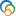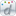The Library of Congress > Linked Data Service > LC Subject Headings (LCSH)

# Ricci flow

• ### Variants

• Flow, Ricci

• ### Closely Matching Concepts from Other Schemes

•Ricci flow•Ricci, Flot de• ### Sources

• found: Work cat.: 2004046148: The Ricci flow, c2004:CIP pref. (the Ricci flow is the geometric evolution equation in which one starts with a smooth Riemannian manifold and evolves its metric)
• found: MathWorld, Mar. 8, 2004(The Ricci flow equation is the evolution equation, d/dt(g)=-2Rc, for a Riemannian metric (g), where Rc is the Ricci curvature tensor. Hamilton (1982) showed that there is a unique solution to this equation for an arbitrary smooth metric on a closed manifold over a sufficiently short time. Hamilton (1982, 1986) also showed that Ricci flow preserves positivity of the Ricci curvature tensor in three dimensions and the curvature operator in all dimensions)
• notfound: CRC concise encyc. math.;Encyc. dict. math.;Math. subj. classif.
• ### Change Notes

• 2004-04-08: new